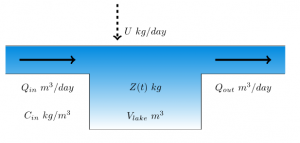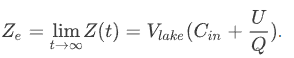# 1.7 Validation

Course subject(s) 1. Introducing Mathematical Modelling

In this section, we first summarise the results up till now for you, then you answer the question of the factory, and finally, you will predict what happens when the situation is reversed.

For your convenience: a summary of the results up till now:A factory wants to start discharging waste material in a lake. The river that feeds into the lake is already polluted somewhat. How much would this new contamination increase the pollution in the lake?

A mathematical model for Z(t), the mass of the pollutant in the lake, when the factory starts discharging a pollutant at t=0:The solution Z(t) will increase toTo quantify the pollution increase caused by the factory, we define:

Ce: The concentration of the pollution in the lake for t→∞ when the factory discharges U kilo of pollutant per day.

C0: The final concentration of the pollution in the lake for t→∞ when the factory does not pollute the lake.

The waste of the factory increases the pollution concentration in the lake with the factor:  Ce/C0.